# 3Ampere’s experiments

Оценка 4.7
docx
07.05.2020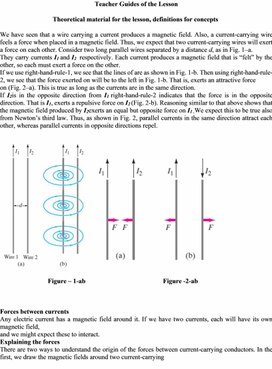3Ampere’s experiments.docx

Teacher Guides of the Lesson

Theoretical material for the lesson, definitions for concepts

We have seen that a wire carrying a current produces a magnetic field. Also, a current-carrying wire feels a force when placed in a magnetic field. Thus, we expect that two current-carrying wires will exert a force on each other. Consider two long parallel wires separated by a distance d, as in Fig. 1–a.

They carry currents I1 and I2  respectively. Each current produces a magnetic field that is “felt” by the other, so each must exert a force on the other.

If we use right-hand-rule-1, we see that the lines of are as shown in Fig. 1-b. Then using right-hand-rule-2, we see that the force exerted on will be to the left in Fig. 1-b. That is, exerts an attractive force

on (Fig. 2–a). This is true as long as the currents are in the same direction.

If I2is in the opposite direction from I1 right-hand-rule-2 indicates that the force is in the opposite direction. That is I1, exerts a repulsive force on I2 (Fig. 2-b). Reasoning similar to that above shows that the magnetic field produced by I2exerts an equal but opposite force on I1 .We expect this to be true also from Newton’s third law. Thus, as shown in Fig. 2, parallel currents in the same direction attract each other, whereas parallel currents in opposite directions repel.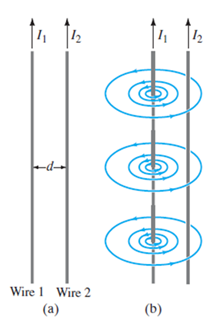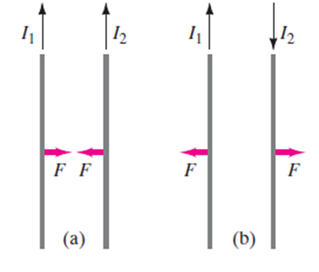Figure – 1-ab                                               Figure -2-ab

Forces between currents

Any electric current has a magnetic field around it. If we have two currents, each will have its own magnetic field,

and we might expect these to interact.

Explaining the forces

There are two ways to understand the origin of the forces between current-carrying conductors. In the first, we draw the magnetic fields around two current-carrying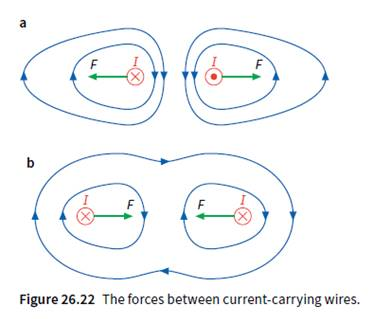conductors (Figure 26.22a). Figure 26.22a shows two unlike (anti-parallel) currents, one flowing into the page, the other flowing out of the page. Their magnetic fields circle round, and in the space between the wires there is an extra-strong field. We imagine the field lines squashed together, and the result is that they push the wires apart.

The diagram shows the resultant field and the repulsive forces on the two wires.

Figure 26.22b shows the same idea, but for two like (parallel) currents. In the space between the two wires, the magnetic fields cancel out. The wires are pushed together. The other way to explain the forces between the currents is to use the idea of the motor effect. Figure 26.24 again shows two like currents, I1 and I2, but this time we only consider the magnetic field of one of them, I1. The second current I2 is flowing across the magnetic field of I1; from the diagram, you can see that B is at right angles to I2. Hence there will be a force on I2 (the BIL force), and we can find its direction using Fleming’s left-hand rule. The arrow shows the direction of the force, which is towards I1. Similarly, there will be a BIL force on I1, directed towards I2.These two forces are equal and opposite to one another.

They are an example of an action and reaction pair, as described by Newton’s third law of motion.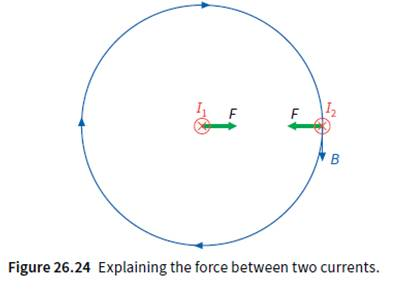Instructions for demonstrations and safety

These activities allow qualitative investigation of the forces on currents.

You will need:

Ø  aluminium foil

Ø  scissors

Ø  large horseshoe magnet

Ø  two clip component holders

Ø  four long 4 mm leads

Ø  power supply, 0–12 V plus rheostat (4 A) (or Westminster Very Low Voltage power supply)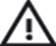 Safety If the current is too large then the foil will get hot and the forces will be too large and tear the foil. When using two strips of foil be aware that if they touch sparking may occur. Care is needed, and the pd must remain modest

You will be making some general observations about forces on currents. As force is a vector, you will be interested to see how large the force is and in what direction it acts. Systematic observations will be essential.

The main point is that currents experience forces in a magnetic field, which can be due to either a permanent magnet or another current. The size of the force depends on the sizes of the current and the magnetic field.  Depending on your circumstances this may well be revision of pre-16 level work. You might like to extend the tasks. How does the force between currents depend on distance? What happens when alternating current is put through the foil in the field? What has this got to do with a possible loudspeaker or microphone? Safety If the current is too large then the foil will get hot and the forces will be too large and tear the foil. When using two strips of foil be aware that if they touch sparking may occur. Care is needed, and the p.d must remain modest

Technician's note:

Westminster power supplies are ideal for the first two experiments. If these are not available it may be necessary to put a high-current rheostat in the circuit to prevent the cut-out operating.

Additional guidelines for organizing a lesson

1.      Organization moment. Establishing emotional state.  Checking for absent students.

2.      Teacher introduces the topic and objectives of the lesson, assess criteria.

3.      Teacher demonstrates students how current-carrying conductor creates a magnetic field

around them and this magnetic field interacts with an external magnetic field, giving rise to a force on the conductor. As the current in the copper rod is switched on, the rod starts to roll, showing that a force is acting on it. Why?

4.      Teacher explains that the production of this magnetic force is known as the motor effect that is why the rod starts to roll, showing that a force is acting on it.

5.      Teacher asks learners in pairs to set up apparatus then observe the attraction and repulsion

between two parallel currents using the equipment. They answer the questions step by step after finishing the experiment.

6.      Students share their knowledge with another pairs, presenting their results in a class. The

teacher together with students make a conclusion.

7.      The study of the new material widely is based on the explanation of the topic by the teacher

accompanied by a presentation.

8. Teacher asks learners individually to answer qualitative questions on the topic of the lesson.

9. At the end of the lesson students are encouraged to reflect on what they have learned and what

they need to improve.

Recommendations for formative assessment

Activity1. Teacher demonstrates students how current-carrying conductor creates a magnetic

field around them and this magnetic field interacts with an external magnetic field

Activity2. Students in pairs set up apparatus then observe the forces between currents.

They answer the questions step by step after finishing the experiment.

Activity3. Individual students were called on to respond to qualitative questions and share their

own opinions/thoughts.

Activity4. At the end of the lesson students are encouraged to reflect on what they have learned

and what they need to improve.

1 – B

2 – C

3 – A

4 – C

5 – A

List of useful links and literature

https://tap.iop.org/fields/electromagnetism/411/page_46911.html

Douglas C. Giancoli, Physics Principles with Applications, Seventh edition 2014.

David Sang, Graham Jones, Gurinder Chadha and Richard Woodside

Cambridge International AS and A Level Course book Second Edition

Скачано с www.znanio.ru

## Figure 26.22a). Figure 26.22a shows two unlike (anti-parallel) currents, one flowing into the page, the other flowing out of the page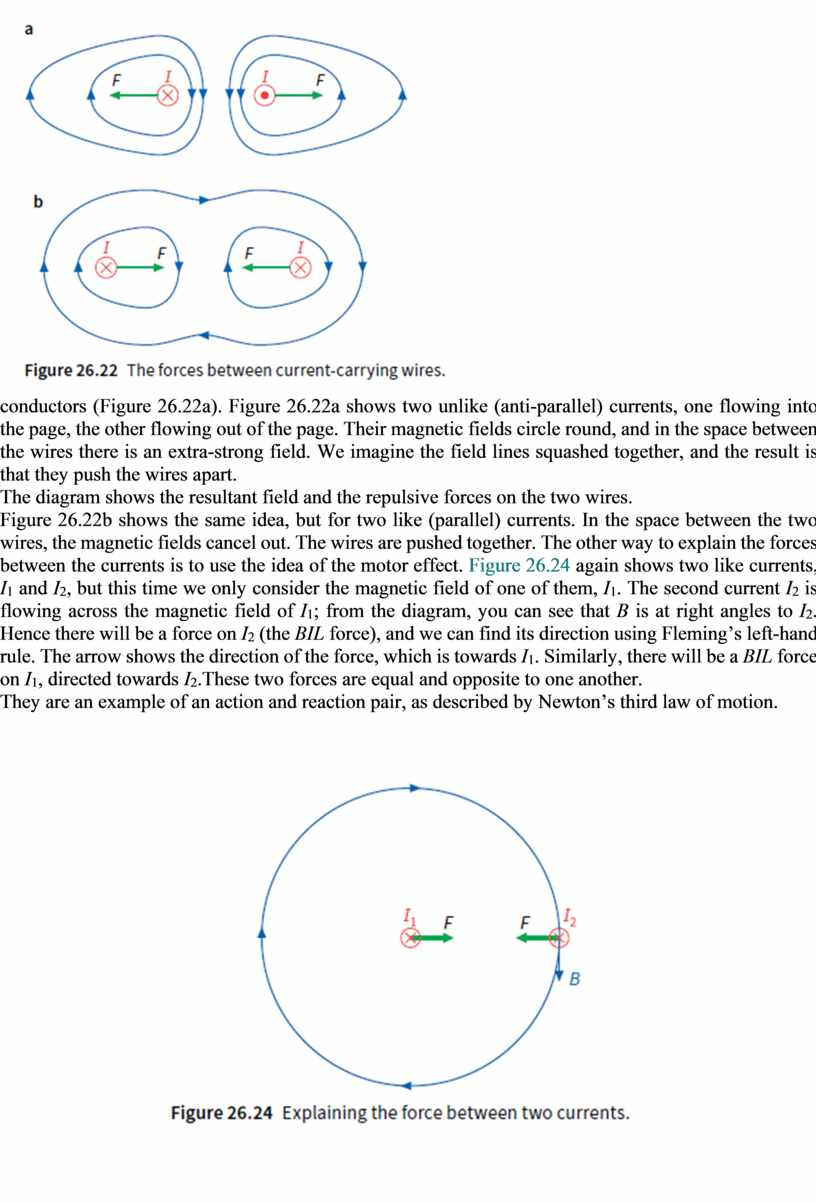Скачать файл